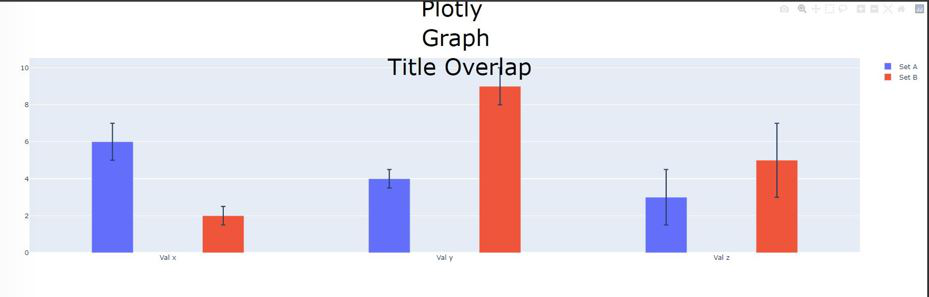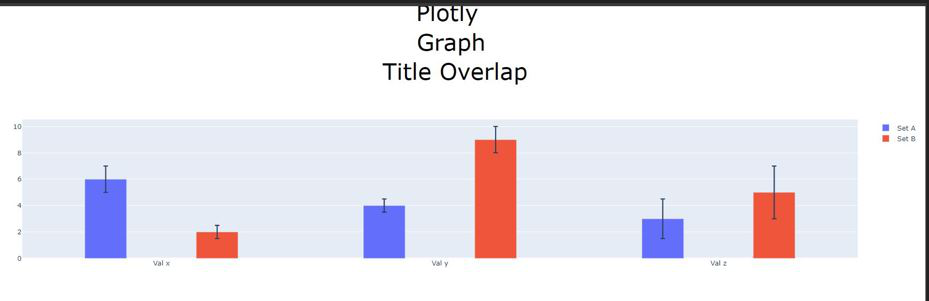Open in App
Not now

# Python Plotly: How to prevent title from overlapping the plot?

• Last Updated : 03 Mar, 2022

The structure & representation of the data are an integral part of the data-driven decision-making process as well as the entire data science pathway. Data View is the process of presenting data in image and image format. Visual representation of data makes understanding complex concepts easier, and new patterns in data can be easily identified. Data in graph or chart format is easy to understand and analyze. Such strategies can help you find the best product development strategy, business growth metrics and other important decisions. Good looking apps are unlimited, can be used to predict sales, stock price analysis, project management, web traffic monitoring, and so on. They are just one example of how digital technology has impacted our daily lives as your car by helping us to better understand it. Plotly’s Python graphing library produces interactive, quality print graphs. Examples of how to create linear sections, scatter sheets, area charts, bar charts, error bars, box sections, histograms, temperature maps, subplots, multiple axes, white charts, and chart bubbles.

Example for Over-Lapping Title

The below python code Creates bar charts for 2 different Categories with 3 elements along the X Axis. The Below Code produces an Output Figure, where the Title overlaps with the Bar plots. There are multiple ways where we can reduce this manually, like Increasing the Figure size or Decreasing the Title Text size . But these are manual methods as different data provides different Bars and we can not manually hard code values.

## Python3

 `import` `plotly.graph_objects as go`   `fig ``=` `go.Figure()` `fig.add_trace(go.Bar(` `    ``name``=``'Set A'``,` `    ``x``=``[``'Val x'``, ``'Val y'``, ``'Val z'``], y``=``[``6``, ``4``, ``3``],` `    ``error_y``=``dict``(``type``=``'data'``, array``=``[``1``, ``0.5``, ``1.5``]),` `    ``width``=``0.15` `))` `fig.add_trace(go.Bar(` `    ``name``=``'Set B'``,` `    ``x``=``[``'Val x'``, ``'Val y'``, ``'Val z'``], y``=``[``2``, ``9``, ``5``],` `    ``error_y``=``dict``(``type``=``'data'``, array``=``[``0.5``, ``1``, ``2``]),` `    ``width``=``0.15` `))` `fig.update_layout(barmode``=``'group'``,` `                  ``title``=``dict``(` `                      ``text``=``"Plotly
Graph
Title Overlap"``,` `                      ``x``=``0.5``,` `                      ``y``=``0.95``,` `                      ``xanchor``=``'center'``,` `                      ``yanchor``=``'top'``,` `                      ``# pad = dict(` `                      ``#            t = 0` `                      ``#           ),` `                      ``font``=``dict``(` `                        `  `                          ``# family='Courier New, monospace',` `                          ``size``=``40``,` `                          ``# color='#000000'` `                      ``)` `                  ``))`     `fig.show()`

Output:Solution :

One Optimal solution is by adjusting the top margin padding. In the below code we are adjusting only the top margin, and setting other margins as default

## Python3

 `import` `plotly.graph_objects as go`   `fig ``=` `go.Figure()`   `# Adding trace for data Set A` `fig.add_trace(go.Bar(` `    ``name``=``'Set A'``,` `    ``x``=``[``'Val x'``, ``'Val y'``, ``'Val z'``], y``=``[``6``, ``4``, ``3``],` `    ``error_y``=``dict``(``type``=``'data'``, array``=``[``1``, ``0.5``, ``1.5``]),` `    ``width``=``0.15` `))` `# Adding trace for data Set B` `fig.add_trace(go.Bar(` `    ``name``=``'Set B'``,` `    ``x``=``[``'Val x'``, ``'Val y'``, ``'Val z'``], y``=``[``2``, ``9``, ``5``],` `    ``error_y``=``dict``(``type``=``'data'``, array``=``[``0.5``, ``1``, ``2``]),` `    ``width``=``0.15` `))`   `# Updating the layout of the figure` `fig.update_layout(barmode``=``'group'``,` `                  ``title``=``dict``(` `                      ``text``=``"Plotly
Graph
Title Overlap"``,` `                      ``x``=``0.5``,` `                      ``y``=``0.95``,` `                      ``xanchor``=``'center'``,` `                      ``yanchor``=``'top'``,` `                      ``# pad = dict(` `                      ``#            t = 0` `                      ``#           ),` `                      ``font``=``dict``(` `                          ``#family='Courier New, monospace',` `                          ``size``=``40``,` `                          ``color``=``'#000000'` `                      ``)` `                  ``))`   `# Now we are setting a top margin of 200, which e` `# enables sufficient space between title and bar` `fig.update_layout(margin``=``dict``(t``=``200``))` `fig.show()`My Personal Notes arrow_drop_up
Related Articles